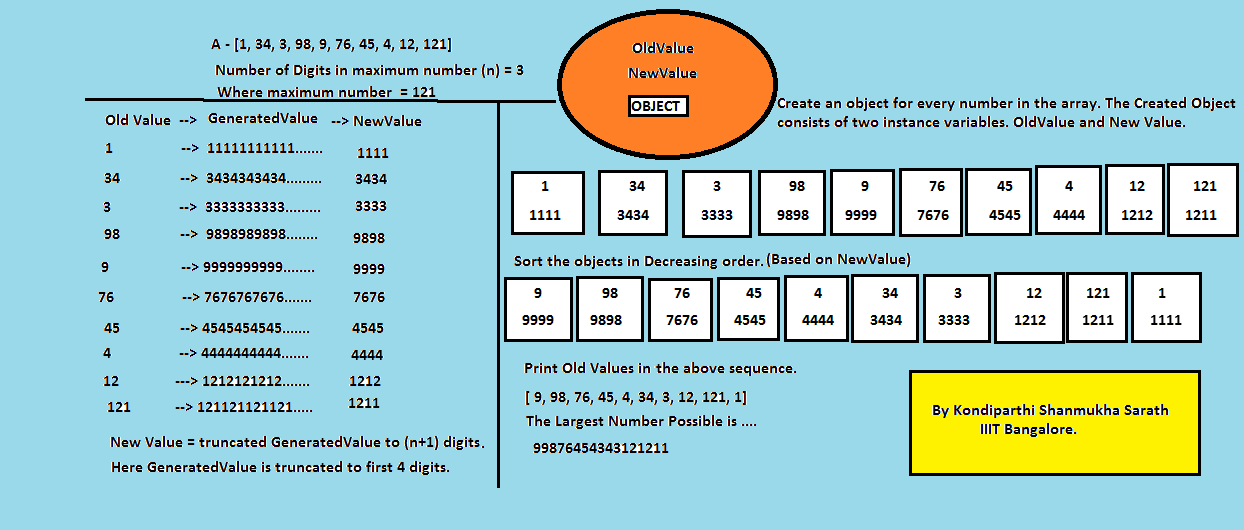# Arrange given numbers to form the biggest number | Set 2

• Difficulty Level : Hard
• Last Updated : 04 Jun, 2020

Given an array of non-negative numbers(of Integer Range), they are needed to be arranged in some order such that it gives the max number. For example given array is A[1, 34, 3, 98, 9, 76, 45, 4, 12, 121]. if we arrange these numbers in the following order, A[9, 98, 76, 45, 4, 34, 3, 12, 121, 1], then by joining them we get “99876454343121211” as largest number.

Examples:

```Input :  [1, 34, 3, 98, 9, 76, 45, 4, 12, 121]
Output : 99876454343121211

Input :  [12, 121]
Output : 12121
```

## Recommended: Please solve it on “PRACTICE ” first, before moving on to the solution.

In SET 1, we have discussed an approach to do same. In this set, we will discuss another logic.

1) Find number of digits in the largest number. Let number of digits be n.
2) Create extended version of all numbers. In extended version, we have n+1 digits formed by concatenating the number of with itself and truncating extra digits.
3) Sort original numbers according to their extended values.
4) Concatenating the sorted numbers produces th required result.## Java

 `// Java program to arrange the numbers to form the ` `// largest number ` `import` `java.math.BigInteger; ` `import` `java.util.*; ` ` `  `public` `class` `LargestNumber ` `{ ` `    ``// method that returns largest number form ` `    ``public` `static` `String largestNumber(List arr) ` `    ``{ ` `        ``// finding number of digits in maximum element ` `        ``// present in array ` `        ``int` `n = ` `         ``Collections.max(arr).toString().length(); ` ` `  `        ``ArrayList en = ` `                       ``new` `ArrayList(); ` `        ``for` `(``int` `i = ``0` `; i < arr.size(); i++) ` `            ``en.add(``new` `ExtendedNum(arr.get(i), ` `                              ``n)); ` ` `  `        ``// sort based on modified value ` `        ``Collections.sort(en, (p1, p2) -> ` `         ``(``int``)(p2.modifiedValue - p1.modifiedValue)); ` ` `  `        ``StringBuilder sb = ``new` `StringBuilder(); ` `        ``for` `(``int` `i = ``0``; i < en.size(); i++) ` `            ``sb.append(``new` `StringBuilder ` `            ``(Long.toString(en.get(i).originalValue))); ` ` `  ` `  `        ``// To remove any zeroes at head. ` `        ``BigInteger bi = ``new` `BigInteger(sb.toString()); ` ` `  `        ``return` `bi.toString(); ` `    ``} ` ` `  `    ``// Driver method ` `    ``public` `static` `void` `main(String[] args) ` `    ``{ ` `        ``Integer arr[] = {``1``, ``34``, ``3``, ``98``, ``9``, ``76``, ``45``, ` `                                     ``4``, ``12``, ``121``}; ` `        ``List l = Arrays.asList(arr); ` ` `  `        ``System.out.println(largestNumber(l)); ` `    ``} ` `} ` ` `  `// A utility class to generate new value ` `class` `ExtendedNum ` `{ ` `    ``int` `originalValue; ` `    ``long` `modifiedValue; ` ` `  `    ``public` `ExtendedNum(``int` `originalValue, ``int` `n) ` `    ``{ ` `        ``this``.originalValue = originalValue; ` `        ``String s = Integer.toString(originalValue); ` `        ``StringBuilder sb = ``new` `StringBuilder(s); ` `        ``StringBuilder ans = ``new` `StringBuilder(); ` `        ``while` `(ans.length() <= n + ``1``) ` `            ``ans.append(sb); ` ` `  `        ``s = ans.toString().substring(``0``, n + ``1``); ` `        ``modifiedValue = Long.parseLong(s); ` `    ``} ` ` `  `    ``public` `String toString() ` `    ``{ ` `        ``return` `"["` `+ modifiedValue + ` `                ``", "` `+ originalValue + ``"]"``; ` `    ``} ` `} `

## Python

 `# Python program to find largest ` `# number from the given values ` `# function that return largest ` `# possible number ` `def` `largestNumber(array): ` `     `  `    ``# extval is a empty list for extended  ` `    ``# values and ans for calculating answer ` `    ``extval, ans ``=` `[], "" ` `     `  `    ``# calculating the length of largest number ` `    ``# from given and add 1 for further operation ` `    ``l ``=` `len``(``str``(``max``(array))) ``+` `1` `     `  `    ``# iterate given values and  ` `    ``# calculating extended values ` `    ``for` `i ``in` `array: ` `        ``temp ``=` `str``(i) ``*` `l ` `         `  `        ``# make tuple of extended value and  ` `        ``# equivalant original value then  ` `        ``# append to list ` `        ``extval.append((temp[:l:], i)) ` `     `  `    ``# sort extval in descending order ` `    ``extval.sort(reverse ``=` `True``) ` `     `  `    ``# iterate extended values ` `    ``for` `i ``in` `extval: ` `         `  `        ``# concatinate original value equivalant ` `        ``# to extended value into ans variable ` `        ``ans ``+``=` `str``(i[``1``]) ` ` `  `    ``if` `int``(ans)``=``=``0``: ` `        ``return` `"0"` `    ``return` `ans ` ` `  `# Driver code ` `a ``=` `[``1``, ``34``, ``3``, ``98``, ``9``, ``76``, ` `        ``45``, ``4``, ``12``, ``121``] ` ` `  `print``(largestNumber(a)) ` ` `  `# This code is contributed by Chhekur `

Output:

```99876454343121211
```

This article is contributed by Kondiparthi Shanmukha Sarath. If you like GeeksforGeeks and would like to contribute, you can also write an article using contribute.geeksforgeeks.org or mail your article to contribute@geeksforgeeks.org. See your article appearing on the GeeksforGeeks main page and help other Geeks.